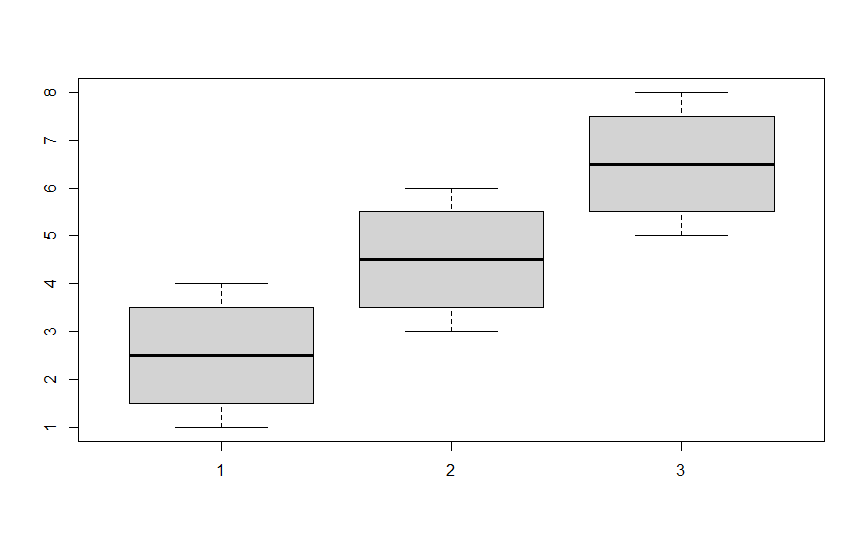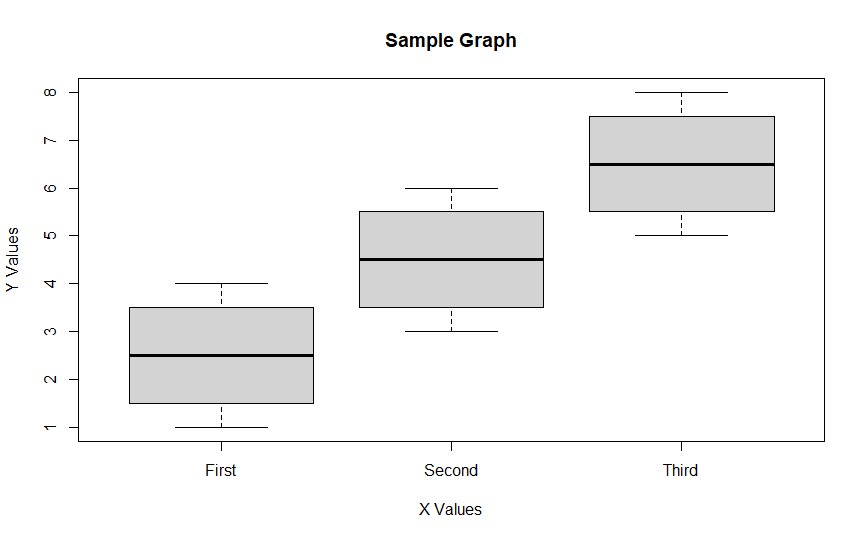# Label BoxPlot in R

R is equipped with many functions for different types of graphs and plots. Such plots are very useful and can provide good insights into the data.

The BoxPlot is a unique and useful graph type. It allows us to study the distribution of data and identify different trends in the dataset. We can use it for comparison between different groups as well.

In R, we generally use the `boxplot()` function to create such graphs but we can also make use of the `geom_boxplot()` function with the `ggplot()` function to create boxplots and there are some other methods available as well.

The following example shows a simple boxplot of three sample distributions using the `boxplot()` function.

``````v1 <- c(1,2,3,4)
v2 <- c(3,4,5,6)
v3 <- c(5,6,7,8)
boxplot(v1,v2,v3)
``````We can also label the graph properly using the right parameters. The `xlab` parameter labels the x-axis and `ylab` parameter labels the y axis. The `main` parameter sets the title of the graph. We can label the different groups present in the plot using the `names` parameter. The following code and graph will show the use of all these parameters.

``````boxplot(v1,v2,v3, main = "Sample Graph",
xlab = "X Values", ylab = "Y Values",
names = c("First","Second","Third"))
``````Notice the difference in both the graphs with the addition of labels in the second graph.

We can also add the `notch` parameter and set it to `TRUE` to add a notch to the boxes and help in studying the medians of data. To make the boxes on the other axis, we can set the `horizontal` parameter to be `TRUE`.

``````boxplot(v1,v2,v3, main = "Sample Graph",
xlab = "X Values", ylab = "Y Values",
names = c("First","Second","Third"), notch = TRUE, horizontal = TRUE)
``````